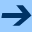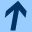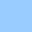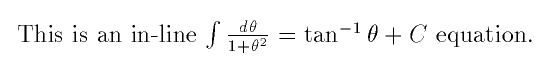In-line equations

### In-line equations

For example, here are three essentially equivalent ways to code in LaTeX the same anti-derivative formula from calculus as an in-line equation. In the first case, mathematics mode is delimited by dollar signs.

This is an in-line $\int \frac{d\theta}{1+\theta^2} = \tan^{-1}\theta+C$ equation.


In the next case, mathematics mode is delimited by the $$ and $$ pair.

This is an in-line $$\int\frac{d\theta} {1+\theta^2}= \tan^{-1} \theta+C$$ equation.


In the third case, mathematics environment is delimited by the \begin{math} and \end{math} pair.

This is an in-line \begin{math}\int\frac
{d\theta}{1+\theta^2} = \tan^{-1}
\theta+ C\end{math} equation.


The advantage of using a dollar sign to delimit mathematics mode is that it is easy to type. On the other hand, using different opening and closing delimiters facilitates error detection and correction.

The output, as formatted by LaTeX, looks like this:• LaTeX ignores input spaces in mathematics mode. (It spaces formulas according to its own internal rules.)

• Standard mathematical functions have natural LaTeX control sequences: \sin, \cos, and so on give the trigonometric functions;  \int makes an integral sign; \frac builds a fraction whose numerator and denominator are the subsequent two items delimited by curly braces.

• The LaTeX control sequences for Greek letters are their usual names preceded by a backslash. For example, \gamma produces a gamma, and \Gamma produces a capital gamma.

• LaTeX uses curly braces for grouping and for delimiting arguments; the braces do not print. (If you really want to make LaTeX print an opening brace, type \{ in your input file.)

• A caret ^ makes a superscript, and an underscore _ makes a subscript.The Math 696 course pages were last modified April 5, 2005.In-line equations